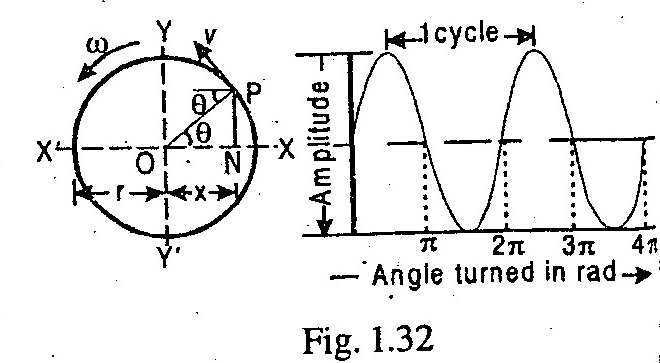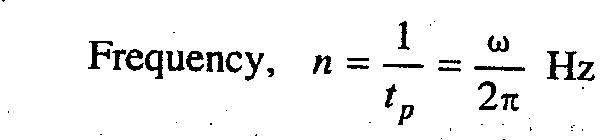## Featured Post

### Best Schools for Mechanical Engineering Degree | University Ranking

Interested in studying from top mechanical engineering schools and institutes in the world? We have compiled a list of best mechanical engin...### Simple Harmonic Motion

What is Simple Harmonic Motion (SHM)? Amplitude, Frequency and Periodic Time of a body moving with SHM?

A body is said to move or vibrate with simple harmonic motion (briefly written as S.H.M.), if it satisfies the following two conditions:

1. Its acceleration is always directed towards the center known as point of reference or mean position, and

2. Its acceleration is proportional to the distance from that point.The following terms are commonly used in simple harmonic motion :

1. Amplitude. It is the maximum displacement of a body from its mean position. In Fig. 1.32, OX and OX' is the amplitude of particle P. The amplitude is always equal to the radius of the circle.

2. Periodic time. It is the time taken for one complete revolution of the particle. Mathematically,3. Frequency. It is the number of cycles per second and it is the reciprocal of time period (tp). Mathematically,Note : In S.I. units, the unit of frequency is hertz (briefly written as Hz) which is equal to one cycle per second.